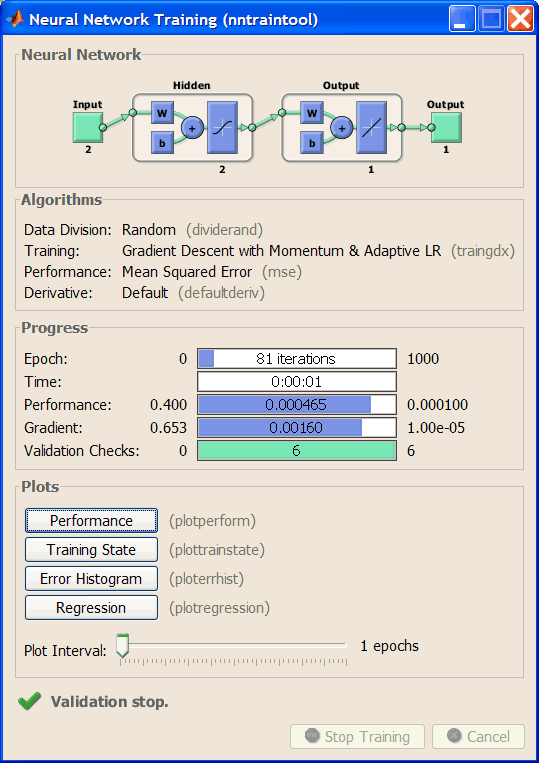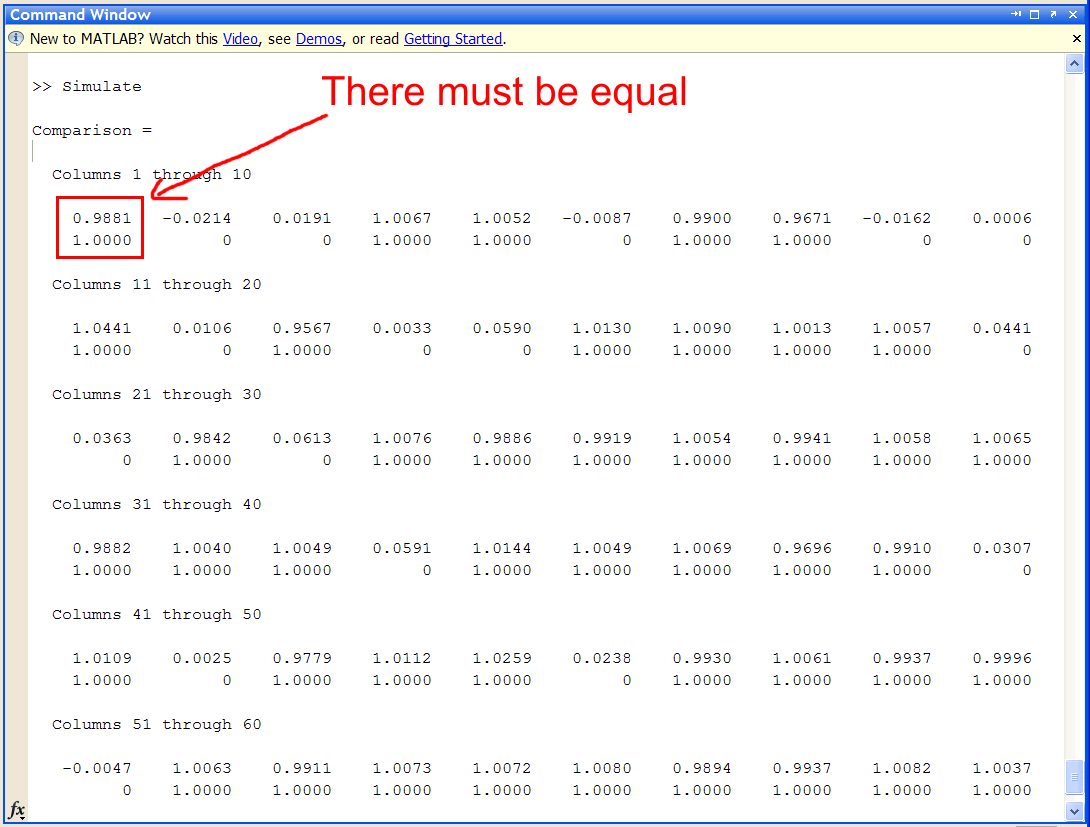# Logic Operation OR using Matlab

 x1 x2 z 0 0 0 0 1 1 1 0 1 1 1 1

 Problem 1 Write the BuildTrainSet.m file using Matlab to build an appropriate training set for the OR operation. Use 64 training cases; observe that each training case is a column in the matrix. (To keep the files organized, create the OrMapMatlab folder for all problems in this section.)

 BuildTrainSet.m clear; numCases=64; % ______________________ Create two random inputs input0=round(rand(1, numCases)); input1=round(rand(1, numCases)); %_______________________ Contaminate the input with noise noisyInput0 = input0*0.9 + rand(1, numCases)*0.1; noisyInput1 = input1*0.9 + rand(1, numCases)*0.1; %________________________ Compute the input and the target trainSetInput = [noisyInput0  ; noisyInput1 ] trainSetTarget = input0 | input1;

 Output > > BuildTrainSet trainSetInput =   Columns 1 through 10     0.0550    0.9145    0.9853    0.0622    0.9351    0.0513    0.0402    0.9076    0.9240    0.9123     0.9812    0.0533    0.0351    0.0939    0.9876    0.0550    0.0622    0.9587    0.9208    0.9301   Columns 11 through 20     0.9184    0.0240    0.9417    0.9050    0.9903    0.9945    0.9491    0.0489    0.9338    0.0900     0.9471    0.0230    0.9844    0.9195    0.0226    0.9171    0.0228    0.0436    0.9311    0.9923   Columns 21 through 30     0.9369    0.0111    0.0780    0.0390    0.0242    0.9404    0.9096    0.0132    0.9942    0.0956     0.9430    0.0185    0.9905    0.0980    0.0439    0.0111    0.0258    0.9409    0.0595    0.9262   Columns 31 through 40     0.0575    0.0060    0.9235    0.9353    0.0821    0.0015    0.0043    0.9169    0.9649    0.9732     0.0603    0.9711    0.0222    0.9117    0.9297    0.9319    0.0424    0.0508    0.0086    0.9262   Columns 41 through 50 ...

 Problem 2 Write the BuildValidSet.m file using Matlab to build a validation set for the OR operation. Use 128 validation cases.

 BuildValidSet.m numCases=128; % ______________________ Create two random inputs input0=round(rand(1, numCases)); input1=round(rand(1, numCases)); %_______________________ Contaminate the input with noise noisyInput0 = input0*0.9 + rand(1, numCases)*0.1; noisyInput1 = input1*0.9 + rand(1, numCases)*0.1; %________________________ Compute the input and the target validSetInput = [noisyInput0  ; noisyInput1 ] validSetTarget = input0 | input1;

 Output > > BuildValiSet validSetInput =   Columns 1 through 10     0.0366    0.9764    0.0628    0.9772    0.0933    0.9973    0.0192    0.9139    0.9696    0.9094     0.9147    0.0189    0.0043    0.0635    0.0282    0.0539    0.0695    0.0499    0.9536    0.0445   Columns 11 through 20     0.9525    0.0530    0.9861    0.0485    0.0393    0.9671    0.9741    0.0520    0.9348    0.9150     0.9124    0.9490    0.9853    0.0874    0.0270    0.0208    0.9565    0.9640    0.0417    0.9206   Columns 21 through 30     0.9586    0.9262    0.9044    0.9755    0.0243    0.0442    0.9688    0.0359    0.0736    0.0395     0.9948    0.9082    0.9106    0.0142    0.0166    0.9621    0.9574    0.0052    0.0931    0.0729   Columns 31 through 40

 Problem 3 Write the Trainx.m file using Matlab to design and train an ANN for the AND gate. Use one hidden layer and one neuron in this layer. Train the ANN using the Conjugate Gradient method:      Number of iterations = 1000      Goal (desired mse) = 0.0001 Note: feedforward is a command available starting on version 2010b. In previous versions, you may use newff.

 Trainx.m %________________________ After version 2010a net = feedforwardnet(2, 'traingdx'); net.trainParam.epochs=1000; net.trainParam.goal=0.0001; net = train(net, trainSetInput, trainSetTarget); %________________________ version 2010a and earlier %ranges = [0,1; %Range of input 0 is from 0 to 1 %    0 1];      %Range of input 1 is from 0 to 1 %net = newff(ranges, [2, 1], {'logsig', 'logsig'}, 'traingdx'); %net.trainParam.epochs=1000; %net.trainParam.goal=0.0001; %net = train(net, trainSetInput, trainSetTarget);Problem 4 Write the CheckTraining.m file using Matlab to check the training by computing the mean squared error for the ANN using the training set.

 CheckTraining.m %________________________ 2010a and later output = net(trainSetInput); mse(output - trainSetTarget) %________________________ Before version 2010a %output = sim(net, trainSetInput); %mse(output - trainSetTarget)

 Output > > CheckTraining ans =   5.0398e-004

 Problem 5 Write the Validation.m file using Matlab to perform the validation of the ANN by computing the mean squared error for the ANN using the validation set.

 Validation.m %________________________ 2010a and later output = net(validSetInput); mse(output - validSetTarget) %________________________ Before version 2010a %output = sim(net, validSetInput); %mse(output - validSetTarget)

 Output > > Validation ans =   6.5930e-004

 Problem 6 Write the Simulatex.m file using Matlab to simulate the ANN previously trained. Observe that in the comparison the elements in each column must be equal.

 Simulatex.m %________________________ 2010a and later output = net(trainSetInput); comparison = [output; trainSetTarget] %________________________ Before version 2010a %output = sim(net, trainSetInput); %comparison = [output; trainSetTarget]